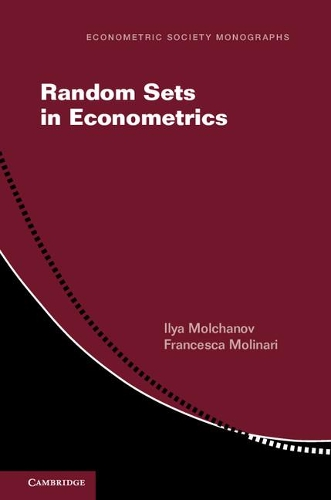•Econometric Society Monographs: Random Sets in Econometrics Series Number 60 (Paperback)

(author), (author)
£26.99
Paperback 194 Pages / Published: 05/04/2018
• We can order this

Usually dispatched within 2 weeks

Random set theory is a fascinating branch of mathematics that amalgamates techniques from topology, convex geometry, and probability theory. Social scientists routinely conduct empirical work with data and modelling assumptions that reveal a set to which the parameter of interest belongs, but not its exact value. Random set theory provides a coherent mathematical framework to conduct identification analysis and statistical inference in this setting and has become a fundamental tool in econometrics and finance. This is the first book dedicated to the use of the theory in econometrics, written to be accessible for readers without a background in pure mathematics. Molchanov and Molinari define the basics of the theory and illustrate the mathematical concepts by their application in the analysis of econometric models. The book includes sets of exercises to accompany each chapter as well as examples to help readers apply the theory effectively.

Publisher: Cambridge University Press
ISBN: 9781107548732
Number of pages: 194
Weight: 310 g
Dimensions: 228 x 153 x 13 mm

MEDIA REVIEWS
'The book builds upon and greatly elaborates on the pioneering, fundamental work that Arie Beresteanu, Ilya Molchanov, and Francesca Molinari did on connecting econometric models with missing data and set-valued responses - such as games with multiple equilibria - to the theory of random sets, a beautiful field of mathematics, probability theory, and mathematical economics. It should be of equal interest to students and researchers in mathematical statistics, econometrics, and machine learning, particularly in problems where set-valued predictions arise, in either observational or counterfactual settings.' V. Chernozhukov, Economics Department and Center for Statistics and Data Science, Massachusetts Institute of Technology
'This book will be very useful to graduate students, faculty, and other researchers interested in the exciting new area of partial identification. Partial identification analysis has become important in empirical economics, largely motivated by revealed preference ideas. Random sets provide a useful and important way of approaching these problems. They are particularly helpful in obtaining sharp identified sets, e.g. ones that are as small as possible. This book is timely and useful in providing an introduction to this new area that is clear and gives the necessary mathematical background for a good understanding of and research on these methods.' Whitney Newey, Ford Professor of Economics, Massachusetts Institute of Technology
'Random set theory provides the natural abstract mathematical language for study of partial identification. Molchanov and Molinari, a brilliant collaboration of mathematician and econometrician, present the fundamentals meticulously and describe the fruitful applications to date. Their book provides the foundation for much more to come.' Charles F. Manski, Board of Trustees Professor in Economics, Northwestern University, Illimois
`The book builds upon and greatly elaborates on the pioneering, fundamental work that Arie Beresteanu, Ilya Molchanov, and Francesca Molinari did on connecting econometric models with missing data and set-valued responses - such as games with multiple equilibria - to the theory of random sets, a beautiful field of mathematics, probability theory, and mathematical economics. It should be of equal interest to students and researchers in mathematical statistics, econometrics, and machine learning, particularly in problems where set-valued predictions arise, in either observational or counterfactual settings.' V. Chernozhukov, Economics Department and Center for Statistics and Data Science, Massachusetts Institute of Technology
`This book will be very useful to graduate students, faculty, and other researchers interested in the exciting new area of partial identification. Partial identification analysis has become important in empirical economics, largely motivated by revealed preference ideas. Random sets provide a useful and important way of approaching these problems. They are particularly helpful in obtaining sharp identified sets, e.g. ones that are as small as possible. This book is timely and useful in providing an introduction to this new area that is clear and gives the necessary mathematical background for a good understanding of and research on these methods.' Whitney Newey, Ford Professor of Economics, Massachusetts Institute of Technology
`Random set theory provides the natural abstract mathematical language for study of partial identification. Molchanov and Molinari, a brilliant collaboration of mathematician and econometrician, present the fundamentals meticulously and describe the fruitful applications to date. Their book provides the foundation for much more to come.' Charles F. Manski, Board of Trustees Professor in Economics, Northwestern University, Illimois